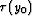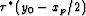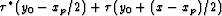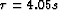Next: HEMNO Implementation Issues Up: Particular Implementation of LSJIMP Previous: Estimation/Application of Seabed Reflection

## HEMNO: Imaging of pegleg multiples in a heterogeneous earth

In Sections-I developed a methodology, valid in a laterally-homogenous earth, to image pegleg multiples and make their amplitudes comparable to their primary. From Figure, recall that a first-order pegleg consists of two unique arrivals: the event with a multiple bounce over the source (source-side'' pegleg), and the event with a bounce over the receiver (receiver-side'' pegleg). When reflectors dip, the arrivals have different traveltimes on a CMP gather, and split'' into two separate events. Figureillustrates the splitting phenomenon on the 2-D Gulf of Mexico dataset which will be used extensively in Chapter. The strong events in the crosshairs'' of the Figure are the first-order source-side and receiver-side seabed pegleg multiples from the top of salt reflection.gulf.split
Figure 6
Splitting phenomenon observed on 2-D prestack field data from the Gulf of Mexico (used in Chapter). The seabed pegleg from the top of salt reflection splits noticeably into two distinct events (labels 1'' and 2''). On the CMP gather the apexes of the two legs are shifted away from zero offset.

On CMP gathers, primary reflection hyperbolas generally have apexes at zero offset. Levin and Shah (1977) show that the apexes of split pegleg multiples shift away from zero offset, raising the apparent velocity of one leg (on one-sided marine data), which can hamper velocity analysis and Radon multiple suppression. Hargreaves et al. (2003) use an apex-shifted Radon transform for demultiple.

Even if reflectors dip only mildly, a prestack imaging method for peglegs must account for splitting. Mild variations in reflector depth over a cable length can introduce considerable destructive interference between the legs of a pegleg multiple at far offsets. This interference, is impossible to model with a 1-D theory, introduces false amplitude-versus-offset (AVO) behavior in the multiples, and certainly would hamper any attempts to use multiples as a constraint on the AVO signature of the primaries Levin (1996).

Levin and Shah (1977) deduced analytic kinematic moveout equations for split 2-D pegleg multiples, and Ross et al. (1999) extended the work to 3-D. Both approaches assume constant velocity and locally planar reflectors - depending on local geology, this may be unrealistic in practice. In this thesis, my particular implementation of LSJIMP relies on a simplified moveout equation based upon a more practically realizable conceptual model, which I call HEMNO (Heterogeneous Earth Multiple NMO Operator). In AppendixI prove that for small reflector dips, the HEMNO traveltime equation reduces to Levin and Shah's moveout equations.schem-pegleg-dip2
Figure 7
HEMNO operator schematic. (a) True raypath in constant-velocity earth. The zero-offset traveltimes to the seabed and subsea reflector areand, respectively. (b) Assumed reflection points under flat-earth assumption. xp is defined in equation (). (c) Stretch legs of raypath vertically to match measuredand. Panel (d) Connect legs of raypath. The solid line that connects the reassembled raypath is the final result.

Figuregraphically illustrates the HEMNO derivation in a constant-velocity earth. Panel a) illustrates the raypath of the S102G'' pegleg where the multiple generator and target reflector dip. Panel b) illustrates the raypath implied by the 1-D multiple imaging theory derived in Section: the primary leg of the multiple has offset xp [equation ()] and the zero-offset traveltime to both reflectors is spatially invariant. Panel c) illustrates the HEMNO strategy: we account for spatially-variant reflector geometry by vertically stretching the 1-D raypath to match the zero-offset traveltime at the reflection points. However, the solid raypath in panel c) disobeys Snell's Law, so to obtain the final result in panel d), the tails of the legs of the ray are simply connected to produce a valid raypath, which has the equation of a hyperbola with offset x and zero-offset traveltime. We first define for simplicity(26)
and then write the HEMNO equation:(27)

Equation () flattens one leg of a first-order pegleg in offset and shifts the event to the zero-offset traveltime of the primary. By using, we ignore lateral velocity variations, which is consistent with the assumption of small dips. Similar expressions may be derived for other multiple events, but I omit the derivations for brevity. Figureillustrates application of the HEMNO equation to the split top of salt pegleg shown in Figure.gulf.hemno.split
Figure 8
Left: CMP gather extracted from midpoint 9200 m of the Mississippi Canyon data cube shown in Figure. Notice the split top of salt pegleg at. Center and Right: HEMNO equation applied to two splits. Notice how the events are independently focused in time and offset. The images panels have also undergone normalized Snell Resampling, differential geometric spreading correction, and application of the seabed reflection coefficient.Next: HEMNO Implementation Issues Up: Particular Implementation of LSJIMP Previous: Estimation/Application of Seabed Reflection
Stanford Exploration Project
5/30/2004